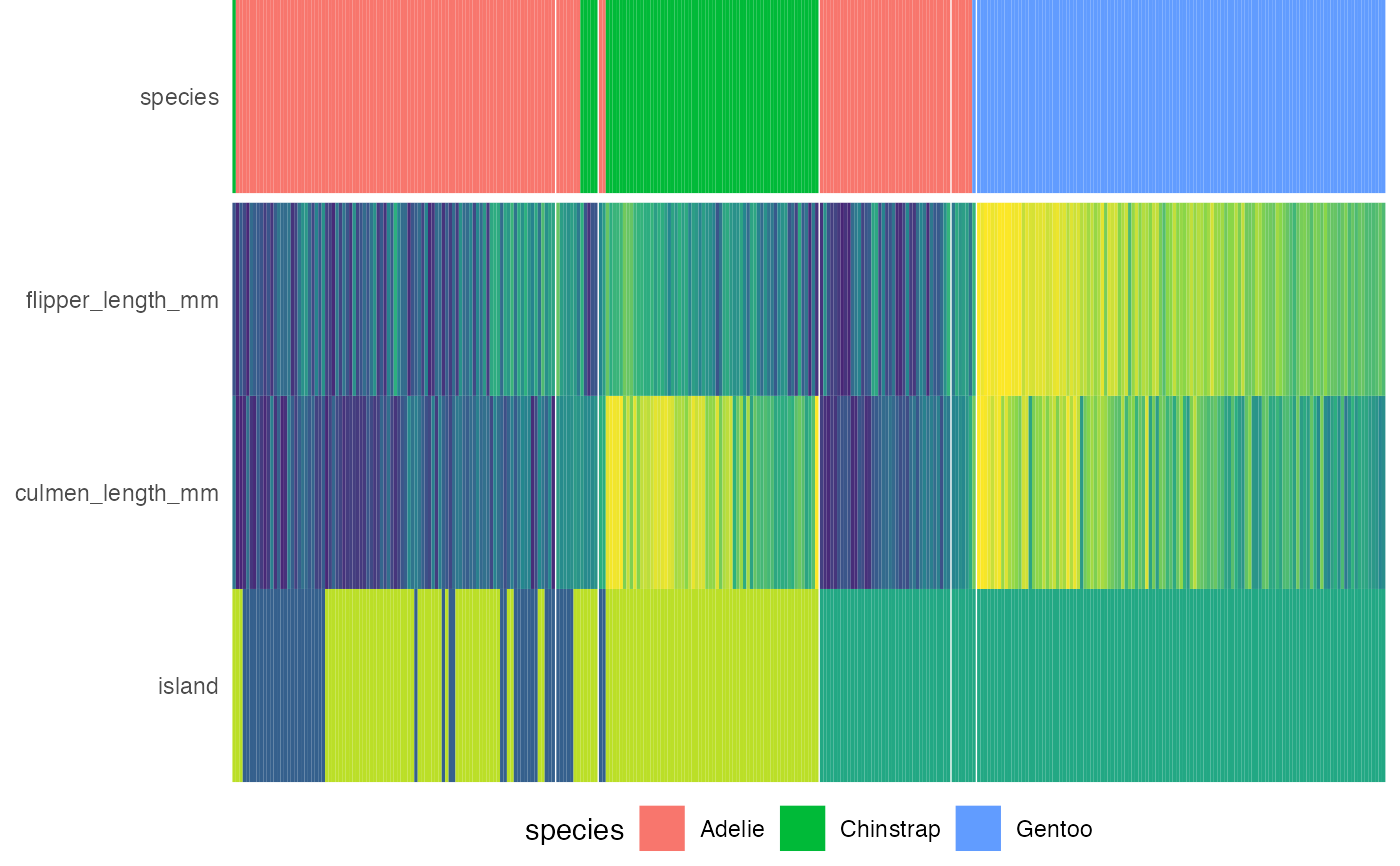Draws the heatmap to be placed below the decision tree.

draw_heat(
dat,
fit,
feat_types = NULL,
target_cols = NULL,
target_lab_disp = fit$target_lab, trans_type = c("percentize", "normalize", "scale", "none"), clust_feats = TRUE, feats = NULL, show_all_feats = FALSE, p_thres = 0.05, cont_legend = "none", cate_legend = "none", cont_cols = ggplot2::scale_fill_viridis_c, cate_cols = ggplot2::scale_fill_viridis_d, panel_space = 0.001, target_space = 0.05, target_pos = "top" ) ## Arguments dat Dataframe with samples from original dataset ordered according to the clustering within each leaf node. fit party object, e.g., as output from partykit::ctree() feat_types Named vector indicating the type of each features, e.g., c(sex = 'factor', age = 'numeric'). If feature types are not supplied, infer from column type. target_cols Character vectors representing the hex values of different level colors for targets, defaults to viridis option B. target_lab_disp Character string for displaying the label of target label. If not provided, use target_lab. trans_type Character string of 'normalize', 'scale' or 'none'. If 'scale', subtract the mean and divide by the standard deviation. If 'normalize', i.e., max-min normalize, subtract the min and divide by the max. If 'none', no transformation is applied. More information on what transformation to choose can be acquired here: https://cran.rstudio.com/package=heatmaply/vignettes/heatmaply.html#data-transformation-scaling-normalize-and-percentize clust_feats Logical. If TRUE, performs cluster on the features. feats Character vector of feature names to be displayed in the heatmap. If NULL, display features of which P values are less than p_thres. show_all_feats Logical. If TRUE, show all features regardless of p_thres. p_thres Numeric value indicating the p-value threshold of feature importance. Feature with p-values computed from the decision tree below this value will be displayed on the heatmap. cont_legend Function determining the options for legend of continuous variables, defaults to FALSE. If TRUE, use guide_colorbar(barwidth = 10, barheight = 0.5, title = NULL). Any other [guides()](https://ggplot2.tidyverse.org/reference/guides.html) functions would also work. cate_legend Function determining the options for legend of categorical variables, defaults to FALSE. If TRUE, use guide_legend(title = NULL). Any other [guides()](https://ggplot2.tidyverse.org/reference/guides.html) functions would also work. cont_cols Function determining color scale for continuous variable, defaults to scale_fill_viridis_c(guide = cont_legend). cate_cols Function determining color scale for nominal categorical variable, defaults to scale_fill_viridis_d(begin = 0.3, end = 0.9). panel_space Spacing between facets relative to viewport, recommended to range from 0.001 to 0.01. target_space Numeric value indicating spacing between the target label and the rest of the features target_pos Character string specifying the position of the target label on heatmap, can be 'top', 'bottom' or 'none'. ## Value A ggplot2 grob object of the heatmap. ## Examples x <- compute_tree(penguins, target_lab = 'species') draw_heat(x$dat, x\$fit)
#> Scale for 'y' is already present. Adding another scale for 'y', which will
#> replace the existing scale.